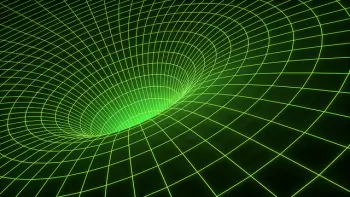# Law of Conservation of Energy: Examples and StatementThe law of conservation of energy states that energy cannot be created or destroyed. The system's energy can only be converted into other types of energy. Therefore, the total energy of an isolated system does not vary with time.

Julius von Mayer enunciated this law.

This law should not be confused with the law of conservation of mass enunciated by Albert Einstein.

The principle of energy conservation has particular views depending on the branch of physics to which we refer. In this article, we refer to five cases:

1. Classical mechanics.

2. Thermodynamics.

3. Dynamics fluids.

4. Electrodynamics.

5. General relativity.

## Law of Conservation of Energy in Classical Mechanics

In Newtonian mechanics, the conservation principle states, "The total mechanical energy of a closed system of bodies, between which only the internal forces of the system act, remains constant.”

Simply put, in the absence of dissipative forces (for example, frictional forces), energy does not come from anywhere and cannot disappear. Instead, it can only be converted into other types of energy.

If dissipative forces appear, such as the friction force, a certain amount of energy is transformed into another form of energy (for example, thermal energy).

## Principle of Conservation of Energy in Thermodynamics

In this context, the law is formulated as the first law of thermodynamics: "The amount of heat received by the system is used to change its internal energy and do work against external forces."

The law of conservation of energy states that there are no perpetual motion machines of the first kind. Sadi Carnot showed that such processes are impossible.

## The Law in Dynamic Fluids

In this dynamics of an ideal fluid, the law of conservation of energy is formulated in the form of the Bernoulli equation: the sum remains constant along the streamlines.

## The Principle in Electrodynamics

In this branch of physics, this law is historically formulated in the Poynting theorem, which relates the density of the flow of electromagnetic energy with the density of electromagnetic energy and the density of Joule's losses.

Verbally, the theorem can be formulated as follows: “A change in electromagnetic energy enclosed in a certain volume over a certain time interval is equal to the flow of this type of energy through the surface that limits a given volume and the amount of thermal energy released in a given volume taken with the opposite sign. ”

## The Law in General Relativity

Being a generalization of the particular theory of relativity, the general theory of relativity uses a generalization of the four-moment concept: the energy-momentum tensor. Hence, the law is formulated for the energy-momentum tensor of the system.

In the general theory of relativity, the law of conservation of energy, strictly speaking, is fulfilled only locally. It is due to the fact that this law is a consequence of the uniformity of time, while in the general theory of relativity, time depends on the presence of bodies and fields in space-time.

## Examples of the Law of Conservation of Energy

To understand how the law of conservation of energy works, we list some examples:

1. A roller coaster car performs various potential and kinetic energy conversions with each turn. For example, almost all the energy is gravitational potential energy when it is at its highest. Then, as it goes down, the speed increases, transforming the energy into kinetic energy.

2. An electric generator can transform mechanical energy into electrical energy.

3. The combustion of a wood fire transforms the chemical energy of the fuel into thermal energy to heat its surroundings.

4. During the melting of the ice, a part of the supplied heat decreases the temperature until it reaches the melting point. From this moment on, the energy flow is used to carry out the phase change from solid to liquid.

5. In a nuclear reaction, atoms lose mass energy which is converted into thermal energy.

Author:

Published: February 10, 2020
Last review: June 30, 2022